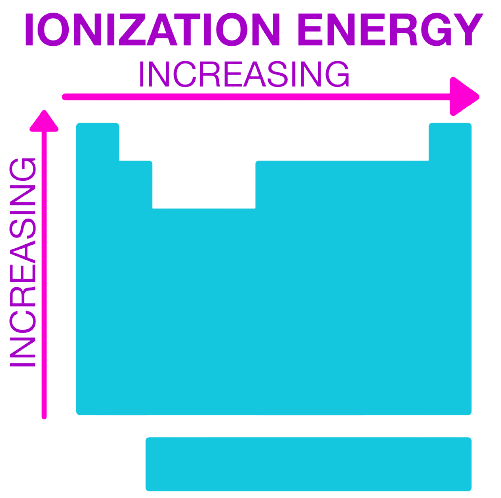# IMAT 2018 Q45 [First Ionisation Energy]

Five atoms are shown below. The letters are NOT chemical symbols.

Which one of the following atoms has the highest first ionisation energy?

A. _{11}^{23}Z

B. _{10}^{20}W

C. _{9}^{21}X

D. _{11}^{22}Y

E. _{9}^{19}V

What is ionization energy?

the energy needed to remove an electron from the ground state of gaseous atoms, ions, or molecules

first ionization energy
minimum energy needed to remove one mole of electrons from one mole of gaseous atoms in their ground state.It increases across a period and decreases down a group. Note that there is a different trend among the transition elements, as per usual.

In this problem, we are given the atomic number and the atomic mass. The atom with the highest first ionization energy will be the one with the element furthest to the right and highest up in the periodic table. We are given the atomic number, and this can tell us where in the periodic table the element is found. The top number is the atomic mass and the bottom number is the atomic number.

Atomic numbers: 9, 10, 11

Going from left to right, it will be 9, 10 and then 11 will actually be in the row below and all the way to the left. Normally this is Sodium (Na), which is a group 1 metal. 9 signifies a halogen and 10 signifies a noble gas. In this case, the noble gas has the highest ionization energy, and this makes sense because it has a stable octet, so it will require the most energy to remove electrons from it.